Select Page

# Maths Continuity and Differentiability CBSE 12 Science MCQ in English Answers

Maths Continuity and Differentiability CBSE 12 Science MCQ in English Answers to enable students to get Answers in a narrative video format for the specific question.

Expert Teacher provides Maths Continuity and Differentiability CBSE 12 Science MCQ English Answers. This video solution will be useful for students to understand how to write an answer in exam in order to score more marks. This teacher uses a narrative style for a question from Continuity and Differentiability not only to explain the proper method of answering question, but deriving right answer too.

Please find the question below and view the Answer in a narrative video format.

Question:

## Similar Questions from CBSE, 12th Science, Maths, Continuity and Differentiability

Question 1 : Findfor the function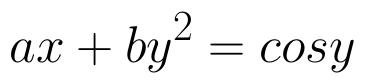. (View Answer Video)

Question 2 :  Differentiate w.r.t.x the function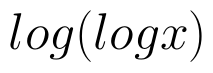. (View Answer Video)

Question 3 : Differentiate the function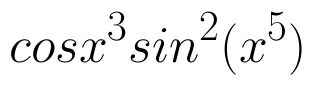with respect to x. (View Answer Video)

Question 4 : Find the value of k, if the area of the triangle is 4 sq unit and vertices are (-2, 0), (0, 4), (0, k). (View Answer Video)

Question 5 :  Find the second order derivative of the function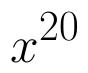. (View Answer Video)

### Relations and Functions

Question 1 : If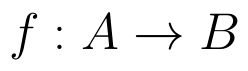and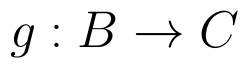are onto, then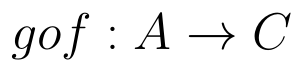is: (View Answer Video)

Question 2 : Letdefined as f(x) = x be an identity function. Then, (View Answer Video)

Question 3 :  A function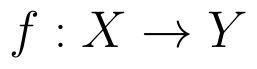is surjective if and only if , (View Answer Video)

Question 4 : Let R be the relation in the set N given by :
R = {(a, b) : a = b - 2, b > 6}.

Question 5 : Let * be the binary operation on N given by a * b = LCM of a and b. Find 20 * 16. (View Answer Video)

### Vector Algebra

Question 1 : Find a vector in the direction of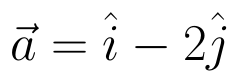that has magnitude 7 units.   (View Answer Video)

Question 2 :  If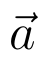and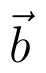denote the position vectors of points A and B respectively and C is a point on AB such that AC = 2 CB, then write the position vector of C.  (View Answer Video)

Question 3 : Find a unit vector parallel to the sum of vectors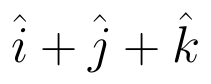and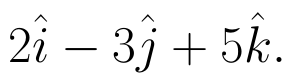(View Answer Video)

Question 4 : If the vertices A, B, C of a triangle ABC have position vectors (1, 2, 3), (-1, 0, 0), (0, 1, 2) respectively then find <ABC (<ABC is the angle between the vectors BA and BC). (View Answer Video)

Question 5 : If a line has direction ratios 2, -1, -2, then what are its direction cosines?   (View Answer Video)

### Three Dimensional Geometry

Question 1 : Find the vector and cartesian forms of the equation of the plane passing through the point (1, 2, -4) and parallel to the lines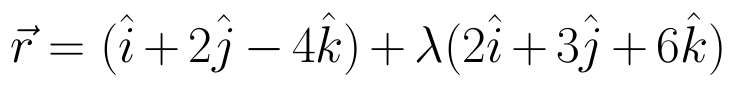and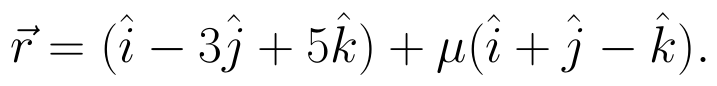Also, find the distance of the point (9, -8, -10) from the plane thus obtained. (View Answer Video)

Question 2 : Find the co-ordinates of the point where the line through the points A(3, 4, 1) and B(5, 1, 6) crosses the XY-plane. Also find angle which this line makes with the XZ-plane. (View Answer Video)

Question 3 : Find the vector equation of a plane which is at a distance of 5 units from the origin and its normal vector is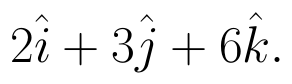(View Answer Video)

Question 4 : Find the direction cosines of the line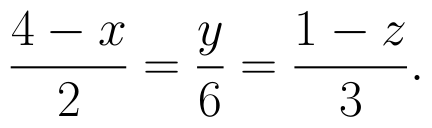(View Answer Video)

Question 5 : Find the equation of the plane passing through the line of intersection of the planes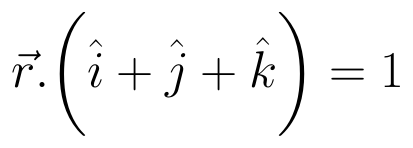and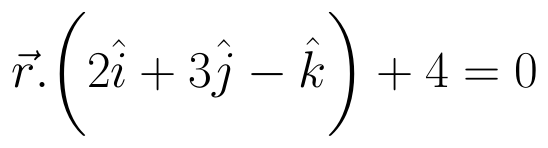which is perpendicular to the plane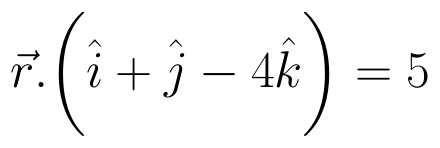. (View Answer Video)